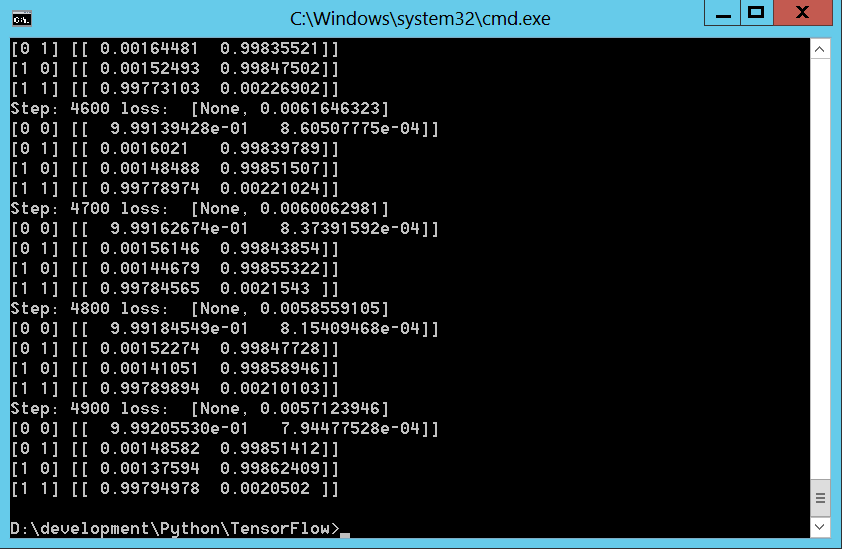用TensorFlow实现AND和XOR 原

#!/usr/bin/env python

import tensorflow as tf
import math
import numpy as np

INPUT_COUNT = 2
OUTPUT_COUNT = 2
HIDDEN_COUNT = 2
LEARNING_RATE = 0.1
MAX_STEPS = 5000

# For every training loop we are going to provide the same input and expected output data
INPUT_TRAIN = np.array([[0, 0], [0, 1], [1, 0], [1, 1]])
OUTPUT_TRAIN = np.array([[1, 0], [0, 1], [0, 1], [1, 0]])

# Nodes are created in Tensorflow using placeholders. Placeholders are values that we will input when we ask Tensorflow to run a computation.
# Create inputs x consisting of a 2d tensor of floating point numbers
inputs_placeholder = tf.placeholder("float",
shape=[None, INPUT_COUNT])
labels_placeholder = tf.placeholder("float",
shape=[None, OUTPUT_COUNT])

# We need to create a python dictionary object with placeholders as keys and feed tensors as values
feed_dict = {
inputs_placeholder: INPUT_TRAIN,
labels_placeholder: OUTPUT_TRAIN,
}

# Define weights and biases from input layer to hidden layer
WEIGHT_HIDDEN = tf.Variable(tf.truncated_normal([INPUT_COUNT, HIDDEN_COUNT]))
BIAS_HIDDEN = tf.Variable(tf.zeros([HIDDEN_COUNT]))

# Define an activation function for the hidden layer. Here we are using the Sigmoid function, but you can use other activation functions offered by Tensorflow.
AF_HIDDEN = tf.nn.sigmoid(tf.matmul(inputs_placeholder, WEIGHT_HIDDEN) + BIAS_HIDDEN)

#  Define weights and biases from hidden layer to output layer. The biases are initialized with tf.zeros to make sure they start with zero values.
WEIGHT_OUTPUT = tf.Variable(tf.truncated_normal([HIDDEN_COUNT, OUTPUT_COUNT]))
BIAS_OUTPUT = tf.Variable(tf.zeros([OUTPUT_COUNT]))

# With one line of code we can calculate the logits tensor that will contain the output that is returned
logits = tf.matmul(AF_HIDDEN, WEIGHT_OUTPUT) + BIAS_OUTPUT
# We then compute the softmax probabilities that are assigned to each class
y = tf.nn.softmax(logits)

# The tf.nn.softmax_cross_entropy_with_logits op is added to compare the output logits to expected output
#cross_entropy = tf.nn.softmax_cross_entropy_with_logits(logits, y)
cross_entropy = -tf.reduce_sum(labels_placeholder * tf.log(y))
# It then uses tf.reduce_mean to average the cross entropy values across the batch dimension as the total loss
loss = tf.reduce_mean(cross_entropy)

# Next, we instantiate a tf.train.GradientDescentOptimizer that applies gradients with the requested learning rate. Since Tensorflow has access to the entire computation graph, it can find the gradients of the cost of all the variables.

# Next we create a tf.Session () to run the graph
init = tf.global_variables_initializer()
with tf.Session() as sess:
# Then we run the session
sess.run(init)

# The following code fetch two values [train_step, loss] in its run call. Because there are two values to fetch, sess.run() returns a tuple with two items. We also print the loss and outputs every 100 steps.
for step in range(MAX_STEPS):
loss_val = sess.run([train_step, loss], feed_dict)
if step % 100 == 0:
print ("Step:", step, "loss: ", loss_val)
for input_value in INPUT_TRAIN:
print (input_value, sess.run(y,
feed_dict={inputs_placeholder: [input_value]}))import tensorflow as tf
import math
import numpy as np

INPUT_COUNT = 2
OUTPUT_COUNT = 2
HIDDEN_COUNT = 2
LEARNING_RATE = 0.1
MAX_STEPS = 5000

# For every training loop we are going to provide the same input and expected output data
INPUT_TRAIN = np.array([[0, 0], [0, 1], [1, 0], [1, 1]])
OUTPUT_TRAIN = np.array([[1, 0], [0, 1], [0, 1], [1, 0]])

# Nodes are created in Tensorflow using placeholders. Placeholders are values that we will input when we ask Tensorflow to run a computation.
# Create inputs x consisting of a 2d tensor of floating point numbers
inputs_placeholder = tf.placeholder("float",
shape=[None, INPUT_COUNT])
labels_placeholder = tf.placeholder("float",
shape=[None, OUTPUT_COUNT])

# We need to create a python dictionary object with placeholders as keys and feed tensors as values
feed_dict = {
inputs_placeholder: INPUT_TRAIN,
labels_placeholder: OUTPUT_TRAIN,
}

# Define weights and biases from input layer to hidden layer
WEIGHT_HIDDEN = tf.Variable(tf.truncated_normal([INPUT_COUNT, HIDDEN_COUNT]))
BIAS_HIDDEN = tf.Variable(tf.zeros([HIDDEN_COUNT]))

# Define an activation function for the hidden layer. Here we are using the Sigmoid function, but you can use other activation functions offered by Tensorflow.
AF_HIDDEN = tf.nn.sigmoid(tf.matmul(inputs_placeholder, WEIGHT_HIDDEN) + BIAS_HIDDEN)

#  Define weights and biases from hidden layer to output layer. The biases are initialized with tf.zeros to make sure they start with zero values.
WEIGHT_OUTPUT = tf.Variable(tf.truncated_normal([HIDDEN_COUNT, OUTPUT_COUNT]))
BIAS_OUTPUT = tf.Variable(tf.zeros([OUTPUT_COUNT]))

# With one line of code we can calculate the logits tensor that will contain the output that is returned
logits = tf.matmul(AF_HIDDEN, WEIGHT_OUTPUT) + BIAS_OUTPUT
# We then compute the softmax probabilities that are assigned to each class
y = tf.nn.softmax(logits)

# The tf.nn.softmax_cross_entropy_with_logits op is added to compare the output logits to expected output
#cross_entropy = tf.nn.softmax_cross_entropy_with_logits(logits, y)
cross_entropy = -tf.reduce_sum(labels_placeholder * tf.log(y))
# It then uses tf.reduce_mean to average the cross entropy values across the batch dimension as the total loss
loss = tf.reduce_mean(cross_entropy)

# Next, we instantiate a tf.train.GradientDescentOptimizer that applies gradients with the requested learning rate. Since Tensorflow has access to the entire computation graph, it can find the gradients of the cost of all the variables.

# Next we create a tf.Session () to run the graph
init = tf.global_variables_initializer()
with tf.Session() as sess:
# Then we run the session
sess.run(init)

# The following code fetch two values [train_step, loss] in its run call. Because there are two values to fetch, sess.run() returns a tuple with two items. We also print the loss and outputs every 100 steps.
for step in range(MAX_STEPS):
loss_val = sess.run([train_step, loss], feed_dict)
if step % 100 == 0:
print ("Step:", step, "loss: ", loss_val)
for input_value in INPUT_TRAIN:
print (input_value, sess.run(y,
feed_dict={inputs_placeholder: [input_value]}))

MNIST的例子可参照如下地址：

https://my.oschina.net/propagator/blog/851912propagator

2018/04/12
424
0
【干货】史上最全的Tensorflow学习资源汇总，速藏！

2018/04/16
0
0
TensorFlow 携手 NVIDIA，使用 TensorRT 优化 TensorFlow Serving 性能

03/07
0
0

2017/01/05
6.1K
10

07/30
0
0

java通过ServerSocket与Socket实现通信

Blueeeeeee
10分钟前
1
0

3
0

12
0

（手机横屏看源码更方便） 注：java源码分析部分如无特殊说明均基于 java8 版本。 简介 大家都知道线程是有生命周期，但是彤哥可以认真负责地告诉你网上几乎没有一篇文章讲得是完全正确的。 ...

13
0
jquery--DOM操作基础

6
0